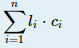odd doctor 已更新

# Deal

Time Limit
8s
Memory Limit
131072KB
Judge Program
Standard
Ratio(Solve/Submit)
33.33%(1/3)
Description:

soda has a tree with n vertices. Each vertex has an initial color with it and the color of i-th vertex is i. And the vitalness of color i is wi. Initially, all the edges have no color and soda wants to color the edges by using the following operation:
1. choose a vertex u and a color c that vertex u contains
2. choose another vertex v and color all the edges and vertices along the shortest path from u to v with color c.

Note that one color will not cover another color, which means one edge and or vertex can have multiply colors.

After m such operations, soda computes the vitalness of the tree as follows:
1. for each color i, find the total length of edges having color i denoting as li
2. the vitalness of the tree isHelp soda find a way to make vitalness of the tree as large as possible after m such operations.

Input:

There are multiple test cases. The first line of input contains an integer T, indicating the number of test cases. For each test case:

The first line contains two integers n and m (1n2000,1m109), the number of vertices and the number of operations.
The following n1 lines describe the edges. The i-th line contains three integer ui, vi and ci (1ui,vin,1ci104) indicating that there is edge between vertex ui and vertex vi and the length is ci.
The next line contains n integers w1,w2,,wn (1wi104), where i-th integer denotes the vitalness of i-th color.

Output:

For each case, output an integer denoting the maximum vitalness soda can get.

Sample Input:
2
3 2
1 2 1
2 3 2
1 10 100
2 100
1 2 1
1 1
Sample Output:
320
2
Source:

2015 Multi-University Training Contest 6

Submit